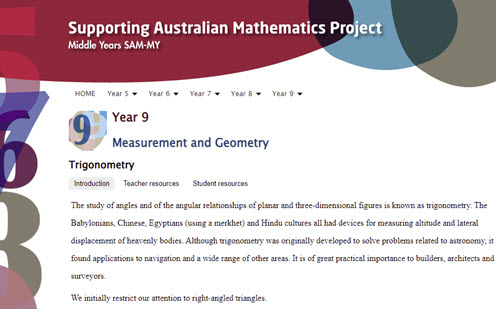Go to website

# Trigonometry

From the AMSI ‘Supporting Australian Mathematics Project’, this resource gives detailed explanations for teachers and students of the curriculum content with worked examples and assessment questions. This resource focuses on the origins of trigonometrical ratios.

Year level(s) Year 9
Audience Teacher
Format Web page
Teaching strategies and pedagogical approaches Explicit teaching
Keywords Pythagoras, Hypotenuse, Adjacent, Opposite, Tangent, Cosine, Sine, Angle, Ratio, Similar, Trigonometry, Right-angled triangle

## Curriculum alignment

Curriculum connections Critical and creative thinking, Numeracy
Strand and focus Space
Topics Pythagoras and trigonometry
AC: Mathematics (V9.0) content descriptions
AC9M9SP02
Apply the enlargement transformation to shapes and objects using dynamic geometry software as appropriate; identify and explain aspects that remain the same and those that change

AC9M9M03

Solve spatial problems, applying angle properties, scale, similarity, Pythagoras' theorem and trigonometry in right-angled triangles

Numeracy progression Proportional thinking (P7)
Understanding geometric properties (P7)
Understanding units of measurement (P10)